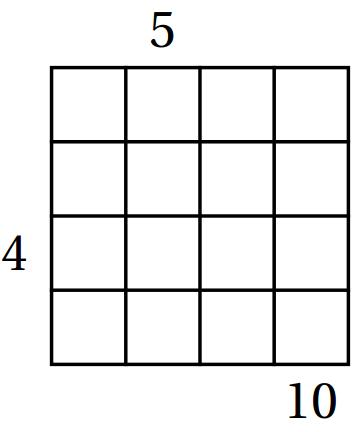## Skyscrapers (Sum)

Skyscraper puzzles are one of my favorite puzzles types. Recently I discovered a new cute variation of this puzzle on the website the Art of Puzzles. But first let me remind you what the skyscraper rules are. There is an n by n square grid that needs to be filled as a Latin square: each number from 1 to n appears exactly once in each row and column. The numbers in the grid symbolize the heights of skyscrapers. The numbers outside the grid represent how many skyscrapers are seen in the corresponding columns/row from the direction of the number.

The new puzzle is called Skyscrapers (Sum), and the numbers outside the grid represent the sum of the heights of the skyscrapers you see from this direction. For example, if the row is 216354, then from the left you see 8(=2+6); and from the right you see 15(=4+5+6).Here’s an easy Skyscrapers (Sum) puzzle I designed for practice.

The Art of Puzzles has four Skyscrapers (Sum) puzzles that are more difficult than the one above:

Share: